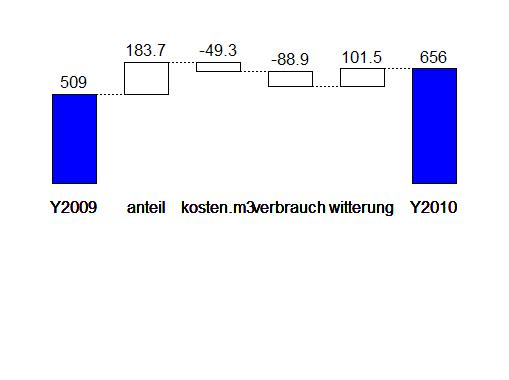## 2011-12-28

### Heating costs

In 2010, my heating costs exceeded my advance payments by about 25%. This motivated me to decompose the costs to see what drove the changes. Here is the result:The numbers refer to Euros. Read von right to left: 2010 was a cold year (+102EUR), but gas consumption in this house was relatively low (–89EUR). Also, running costs and gas price were below previous year’s value (–49EUR). The main driver (+183EUR) for the increased costs was my share on the total costs. In 2009, I had to pay 1.7% of the total costs, which rose to 2.3% in 2010. This means my landlord assumes I used more energy than the other tennants in 2010. I doubt that, and one aspect to support my doubts is that the values of the two heat meters in my living room were nearly at the same value, while I used only one heater.

Thus I believe it was a measurement error. I am not going to complain about this, but will check next measurements very carefully.

Not a direct result of the decomposition, but also interesting is the (temperature adjusted) enery use per square meter, which was in 2010 by about 110 kWh/m2, which is nearly average.

Here’s the R code to produce the chart:

The data is an example data set of my `pft` package, the decomposition is performed with the `ida` package. These packages are not yet on CRAN (I consider them not mature enough). They are available in my personal repository and can be installed with

``````> install.packages(
+   c("ida", "pft"),
+   repos=c(getOption("repos"),
+      "http://userpage.fu-berlin.de/~kweinert/R"),
+   dependencies=c("Depends", "Suggests")
+ )``````

Currently, only R 2.13 and R 2.14 binaries for windows are available.

We represent the heating costs as a product

C(t) = g(t) * w(t) * i(t) * s(t)

with

• g(t), the temperature adjusted gas consumption
• h(t), the relation between the heating degree days in year t and the long term average heating degree days
• i(t), the costs per cbm gas including running costs
• s(t), the share of total costs attributed to me

The dataset `pft.heating` contains these contributing factors, and is loaded with the `data` function. The decomposition is calculated with the `ida` function.

``````> data(pft.heating)
> decomp <- ida(decomp, effect="variable",
+    from="Y2009", to="Y2010",
+    method="lmdi1")``````

The resulting object `decomp` has a custom `plot` method. If the `plotrix` package is installed, it produces the waterfall chart above, otherwise a bar chart.

``> plot(decomp, digits=1)``